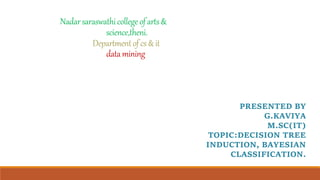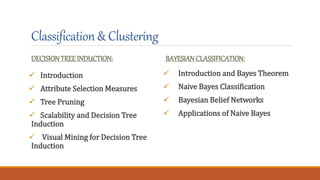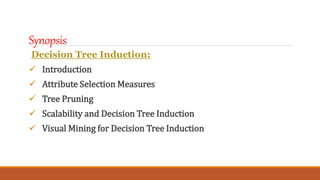Seu SlideShare está sendo baixado. ×
Anúncio
Anúncio
Anúncio
Anúncio
Anúncio
Anúncio
Anúncio
Anúncio
Anúncio
Anúncio
AnúncioCarregando em…3
×

1 de 43 Anúncio

# data mining.pptx

DCOM (Distributed Component Object Model) and CORBA (Common Object Request Broker Architecture) are two popular distributed object models. In this paper, we make architectural comparison of DCOM and CORBA at three different layers: basic programming architecture, remoting architecture, and the wire protocol architecture.

DCOM (Distributed Component Object Model) and CORBA (Common Object Request Broker Architecture) are two popular distributed object models. In this paper, we make architectural comparison of DCOM and CORBA at three different layers: basic programming architecture, remoting architecture, and the wire protocol architecture.

Anúncio
Anúncio

### data mining.pptx

1. 1. Nadarsaraswathicollegeofarts& science,theni. Departmentofcs&it datamining PRESENTED BY G.KAVIYA M.SC(IT) TOPIC:DECISION TREE INDUCTION, BAYESIAN CLASSIFICATION.
2. 2. Classification& Clustering DECISIONTREE INDUCTION:  Introduction  Attribute Selection Measures  Tree Pruning  Scalability and Decision Tree Induction  Visual Mining for Decision Tree Induction BAYESIAN CLASSIFICATION:  Introduction and Bayes Theorem  Naive Bayes Classification  Bayesian Belief Networks  Applications of Naive Bayes
3. 3. Synopsis Decision Tree Induction:  Introduction  Attribute Selection Measures  Tree Pruning  Scalability and Decision Tree Induction  Visual Mining for Decision Tree Induction
4. 4. Decision Tree Induction Introduction:  Decision tree induction is the method of learning the decision trees from the training set. The training set consists of attributes and class labels. Applications of decision tree induction include astronomy, financial analysis, medical diagnosis, manufacturing, and production.  A decision tree is a flowchart tree-like structure that is made from training set tuples. The dataset is broken down into smaller subsets and is present in the form of nodes of a tree. The tree structure has a root node, internal nodes or decision nodes, leaf node, and branches. The root node is the topmost node.
5. 5. Continue: The following decision tree is for the concept buy_computer that indicates whether a customer at a company is likely to buy a computer or not. Each internal node represents a test on an attribute. Each leaf node represents a class.
6. 6. Decision Tree Induction for Machine Learning: ID3 In the late 1970s and early 1980s, J.Ross Quinlan was a researcher who built a decision tree algorithm for machine learning. This algorithm is known as ID3, Iterative Dichotomiser. This algorithm was an extension of the concept learning systems described by E.B Hunt, J, and Marin. ID3 later came to be known as C4.5. ID3 and C4.5 follow a greedy top-down approach for constructing decision trees. The algorithm starts with a training dataset with class labels that are portioned into smaller subsets as the tree is being constructed.
7. 7. Continue: 1) Initially, there are three parameters i.e. attribute list, attribute selection method and data partition. The attribute list describes the attributes of the training set tuples. 2) The attribute selection method describes the method for selecting the best attribute for discrimination among tuples. The methods used for attribute selection can either be Information Gain or Gini Index. 3) The structure of the tree (binary or non-binary) is decided by the attribute selection method. 4) When constructing a decision tree, it starts as a single node representing the tuples.
8. 8. Continue: 5) If the root node tuples represent different class labels, then it calls an attribute selection method to split or partition the tuples. The step will lead to the formation of branches and decision nodes. 6) The splitting method will determine which attribute should be selected to partition the data tuples. It also determines the branches to be grown from the node according to the test outcome. The main motive of the splitting criteria is that the partition at each branch of the decision tree should represent the same class label.
9. 9. An example of splitting attribute is shown below: The portioning above is discrete-valued. The portioning above is for continuous-valued.
10. 10. Continue: 7) The above partitioning steps are followed recursively to form a decision tree for the training dataset tuples. 8) The portioning stops only when either all the partitions are made or when the remaining tuples cannot be partitioned further. 9) The complexity of the algorithm is described by n * |D| * log |D| where n is the number of attributes in training dataset D and |D| is the number of tuples.
11. 11. Why decision tree induction in data mining? – relatively faster learning speed (than other classification methods) – convertible to simple and easy to understand classification rules – can use SQL queries for accessing databases – comparable classification accuracy with other Methods.
12. 12. Advantages Of Decision Tree Classification Enlisted below are the various merits of Decision Tree Classification: 1. Decision tree classification does not require any domain knowledge, hence, it is appropriate for the knowledge discovery process. 2. The representation of data in the form of the tree is easily understood by humans and it is intuitive. 3. It can handle multidimensional data. 4. It is a quick process with great accuracy.
13. 13. Disadvantages Of Decision Tree Classification Given below are the various demerits of Decision Tree Classification: 1. Sometimes decision trees become very complex and these are called overfitted trees. 2. The decision tree algorithm may not be an optimal solution. 3. The decision trees may return a biased solution if some class label dominates it.
14. 14. What Is Greedy Recursive Binary Splitting? In the binary splitting method, the tuples are split and each split cost function is calculated. The lowest cost split is selected. The splitting method is binary which is formed as 2 branches. It is recursive in nature as the same method (calculating the cost) is used for splitting the other tuples of the dataset. This algorithm is called as greedy as it focuses only on the current node. It focuses on lowering its cost, while the other nodes are ignored.
15. 15. How To Select Attributes For Creating A Tree? Attribute selection measures are also called splitting rules to decide how the tuples are going to split. The splitting criteria are used to best partition the dataset. These measures provide a ranking to the attributes for partitioning the training tuples. The most popular methods of selecting the attribute are: Information gain Gain Ratio. Gini index.
16. 16. Information Gain This method the main method that is used to build decision trees. It reduces the information that is required to classify the tuples. It reduces the number of tests that are needed to classify the given tuple. The attribute with the highest information gain is selected. The original information needed for classification of a tuple in dataset D is given by:
17. 17. Continue: Where p is the probability that the tuple belongs to class C. The information is encoded in bits, therefore, log to the base 2 is used. E(s) represents the average amount of information required to find out the class label of dataset D. This information gain is also called Entropy. The information required for exact classification after portioning is given by the formula: Where P (c) is the weight of partition. This information represents the information needed to classify the dataset D on portioning by X.
18. 18. Continue: Information gain is the difference between the original and expected information that is required to classify the tuples of dataset D. Gain is the reduction of information that is required by knowing the value of X. The attribute with the highest information gain is chosen as “best”.
19. 19. Gain Ratio: Information gain might sometimes result in portioning useless for classification. However, the Gain ratio splits the training data set into partitions and considers the number of tuples of the outcome with respect to the total tuples. The attribute with the max gain ratio is used as a splitting attribute.
20. 20. Gini Index: Gini Index is calculated for binary variables only. It measures the impurity in training tuples of dataset D, as P is the probability that tuple belongs to class C. The Gini index that is calculated for binary split dataset D by attribute A is given by: Where n is the nth partition of the dataset D.
21. 21. Continue:  The reduction in impurity is given by the difference of the Gini index of the original dataset D and Gini index after partition by attribute A.  The maximum reduction in impurity or max Gini index is selected as the best attribute for splitting. Overfitting In Decision Trees  Overfitting happens when a decision tree tries to be as perfect as possible by increasing the depth of tests and thereby reduces the error. This results in very complex trees and leads to overfitting.  Overfitting reduces the predictive nature of the decision tree. The approaches to avoid overfitting of the trees include pre pruning and post pruning.
22. 22. What Is Tree Pruning? Tree pruning is the method to reduce the unwanted branches of the tree. This will reduce the complexity of the tree and help in effective predictive analysis. It reduces the overfitting as it removes the unimportant branches from the trees. There are two ways of pruning the tree: 1) Prepruning 2) Postpruning Pre-pruning − The tree is pruned by halting its construction early. Post-pruning - This approach removes a sub-tree from a fully grown tree.
23. 23. Cost Complexity The cost complexity is measured by the following two parameters −  Number of leaves in the tree  Error rate of the tree.
24. 24. Scalability Classifying data sets with millions of examples and hundreds of attributes with reasonable speed. Scalable Decision Tree Induction Methods  SLIQ (EDBT’96 — Mehta et al.) – Builds an index for each attribute and only class list and the current attribute list reside in memory
25. 25. Continue:  SPRINT (VLDB’96 — J. Shafer et al.) – Constructs an attribute list data structure  RainForest (VLDB’98 — Gehrke, Ramakrishnan & Ganti) – Builds an AVC-list (attribute, value, class label)  BOAT (PODS’99 — Gehrke, Ganti, Ramakrishnan & Loh) – Uses bootstrapping to create several small Samples.
26. 26. Scalability Framework for RainForest Separates the scalability aspects from the criteria that determine the quality of the tree  Builds an AVC-list: AVC (Attribute, Value, Class_label) o AVC-set (of an attribute X ) – Projection of training dataset onto the attribute X and class label where counts of individual class label are aggregated. o AVC-group (of a node n ) – Set of AVC-sets of all predictor attributes at the node n.
27. 27. Continue:
28. 28. BOAT (Bootstrapped Optimistic Algorithm for Tree Construction) • Use a statistical technique called bootstrapping to create several smaller samples (subsets), each fits in memory • Each subset is used to create a tree, resulting in several trees • These trees are examined and used to construct a new tree T’ – It turns out that T’ is very close to the tree that would be generated using the whole data set together. • Adv: requires only two scans of DB, an incremental alg.
29. 29. Presentation of Classification Results:
30. 30. Visualization of a Decision Tree in SGI/Mine Set 3.0
31. 31. Interactive Visual Mining by Perception-Based Classification (PBC):
32. 32. SYNOPSIS: Bayesian classification: 1) Introduction and Bayes Theorem 2) Naive Bayes Classification 3) Bayesian Belief Networks 4) Applications of Naive Bayes
33. 33. Introduction ● Bayesian classifiers are the statistical classifiers based on Bayes' Theorem ● Bayesian classifiers can predict class membership probabilities i.e. the probability that a given tuple belongs to a particular class. ● It uses the given values to train a model and then it uses this model to classify new data
34. 34. What is Bayes Theorem? ● Bayes' theorem, named after 18th-century British mathematician Thomas Bayes, is a mathematical formula for determining conditional probability ● The theorem provides a way to revise existing predictions or theories given new or additional evidence. ● In finance, Bayes' theorem can be used to rate the risk of lending money to potential borrowers.
35. 35. Bayes Formula ● The formula for the Bayes theorem is given as follows: ● Bayes' theorem is also called Bayes' Rule or Bayes' Law.
36. 36. What are Bayesian Classifiers? ● Statistical classifiers. ● Predict class membership probabilities, such as the probability that a given tuple belongs to a particular class. ● Based on Bayes’ theorem ● Exhibits high accuracy and speed when applied to large databases.
37. 37. Naive Bayes Classification Before explaining the mathematical representations, let us see the basic principle of Bayesian classification : Predict the most probable class for each instance. How ? Find out the probability of the previously unseen instance belonging to each class, and then select the most probable class.
38. 38. Naive Bayes Classification A Naive Bayes Classifier is a program which predicts a class value given a set of set of attributes. For each known class value, ● Calculate probabilities for each attribute, conditional on the class value. ● Use the product rule to obtain a joint conditional probability for the attributes. ● Use Bayes rule to derive conditional probabilities for the class variable. Once this has been done for all class values, output the class with the highest probability.
39. 39. Model Parameters For the Bayes classifier, we need to “learn” two functions, the likelihood and the prior. each feature is conditionally independent of every other feature for a particular class label. This reduces the number of model parameters.
40. 40. Bayes Classification Naive Bayes works equally well for multi valued attributes also.
41. 41. “Zero” Problem What if there is a class, Ci and X has an attribute Xk such that none of the samples in Ci has that attribute value? In that case P(xk|Ci ) = 0, which results in P(X|Ci ) =0 even though P(xk|Ci ) for all the other attributes in X may be large.